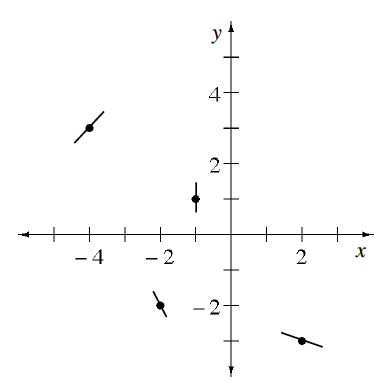### Home > CALC3RD > Chapter Ch7 > Lesson 7.3.2 > Problem7-113

7-113.

Remember Theresa, the girl who loves tangents? Well, Theresa is at it again! She has drawn tangents to a function and then erased the function.

1. Trace her diagram on your paper. Using the tangents, sketch a possible function.

Make sure your sketch of $g\left(x\right)$ goes through all the coordinate points in order AND has the indicated slope at each point.

Recall that a local max or local min COULD exist in one of two places: where the derivative (slope) $= 0$ or where the derivative does not exist.
But, just because $g^\prime(x) = 0$ or $g^\prime(x) = \text{DNE}$, does not mean that that MUST be the location of a local max or local min.